# IBPS Clerk Mains Reasoning Questions 2019 – (Day-9)

Dear Aspirants, Our IBPS Guide team is providing new pattern Reasoning Questions for IBPS Clerk Mains 2019 so the aspirants can practice it on a daily basis. These questions are framed by our skilled experts after understanding your needs thoroughly. Aspirants can practice these new series questions daily to familiarize with the exact exam pattern and make your preparation effective.

“Ensure Your Ability Before of Exam “

## Take IBPS Clerk Free Mock Test 2019 – Start Now

[WpProQuiz 7505]

Input and Output

Directions (1-4): Study the following information to answer the given questions

A number arrangement machine when given an input line of numbers rearranges them following a particular rule. The following is an illustration of input and re-arrangement.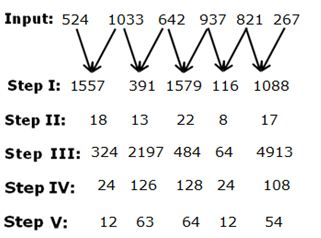Step V is the output of the above input.

As per the rules followed in the above steps, find out in each of the following questions the appropriate steps for the given input.

Input:  317   823   967    1047    1127    198

1) What is the sum of second element from the right end in step I and second element from the left end in step V?

a) 121

b) 143

c) 169

d) 153

e) None of these

2) Which of the following element is immediate left of second to the right of third from the left end in step IV?

a) 108

b) 126

c) 128

d) 24

e) None of these

3) How many elements are there between ‘126’ and ‘9’ in step IV?

s) Three

b) Five

c) Two

d) Four

e) None of these

4) Which of the following step represents the following output?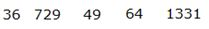a) Step III

b) Step II

c) Step IV

d) There is no such step

e) None of these

Syllogism

Directions (5-7): In the questions below are given some statements followed by some conclusions. You have to choose the conclusion that logically satisfies the given set of statements. Assume the given statements to be true even if they seem to be at variance from commonly known facts.

5) Statements:

All may are june

All june are august

Some june are july

Some july are april

No august is april

Conclusions:

I. Some june are not april is a possibility

II. All july are april is a possibility

III. Some june which are july being april is a possibility

a) None follows

b) Only I and II follow

c) Only II and III follow

d) All follow

e) None of these

Statements:

Some potato are tomato

Some tomato are onion

All tomato are brinjal

No brinjal is capsicum

Conclusions:

I. All capsicum are potato is a possibility

II. Some potato which are tomato being onion is a possibility

III. All onion can never be capsicum

a) Only I and III follow

b) Only II and III follow

c) Only I and II follow

d) All follow

e) None of these

7) Statements:

No pen is pencil

All pencil are ink

No pencil are eraser

All eraser are sharpener

Conclusions:

I. Some ink are sharpener

II. Some pen are eraser is a possibility

III. Some pen which are ink being pencil is a possibility

a) Only III follows

b) Only I and II follow

c) Only II follows

d) All follow

e) None of these

Coding Decoding

Directions (8-10): In each of these questions a group of letters is given and is followed by combinations of number and symbols. Letters are to be coded as per the rules and conditions given below.

The Consonants are coded from 2 to 8 as per the English alphabetical order.

The vowels are coded as >, %, @, !, and # in the same order as per the alphabetical series.

For e.g.

B-2, C-3,D-4…J-8,K-2..R-8 and So on.

So the code for the word ‘SCHOOL’ is ‘3&7&!2’ (after applying the below conditions).

Conditions:

i) If the first and last letter of the word is a consonant, both are to be interchanged.

ii) If the second letter is a consonant and the fourth letter is a vowel, then both are to be coded as &.

iii) If a vowel followed by a vowel, then code for both has to be interchanged.

iv) If more than one vowel (not the same) is present in a word, then third letter is to be coded as ©

Note: More than one condition can apply for the same word.

8) What is the code for “PREDECESSOR COVERT”?

e) None of these

9) What is the code for “SERIAL STRING”?

e) None of these

10) What is the code for “EXPRESSION DOWN”?

e) None of these

Directions (1-4):

Step I: Alternative addition and subtraction of two numbers which is represented by arrow.

Step II: sum of the digits obtained in a number

Step III: Square of even number and cube of odd number

Step IV: Multiplication of each digit in a number

Step V: Each number is divided by 2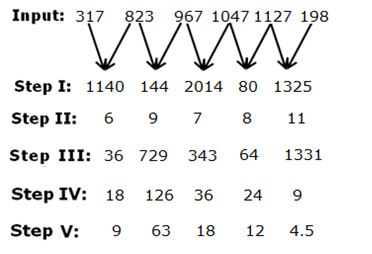Directions (5-7):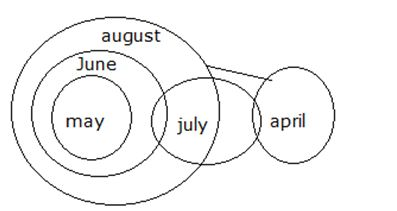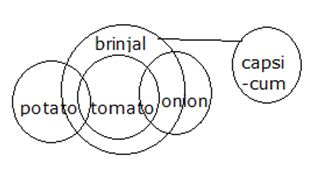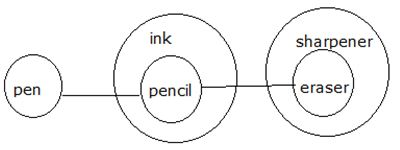Directions (8-10):

A->, B-2, C-3, D-4, E-%, F-5, G-6, H-7, [email protected], J-8, K-2, L-3, M-4, N-5, O-!, P-6, Q-7, R-8, S-2, T-3, U-#, V-4, W-5, X-6, Y-7, Z-8.

Condition (i) and (iv) applies

–> PREDECESSOR

Condition (i) and (iv) applies

–> COVERT

–> SERIAL -Condition (i), (iii) and (iv) applies

–> STRING-Condition (i) and (ii) applies

–>6&8&52

–> EXPRESSION -Condition (iii) and (iv) applies